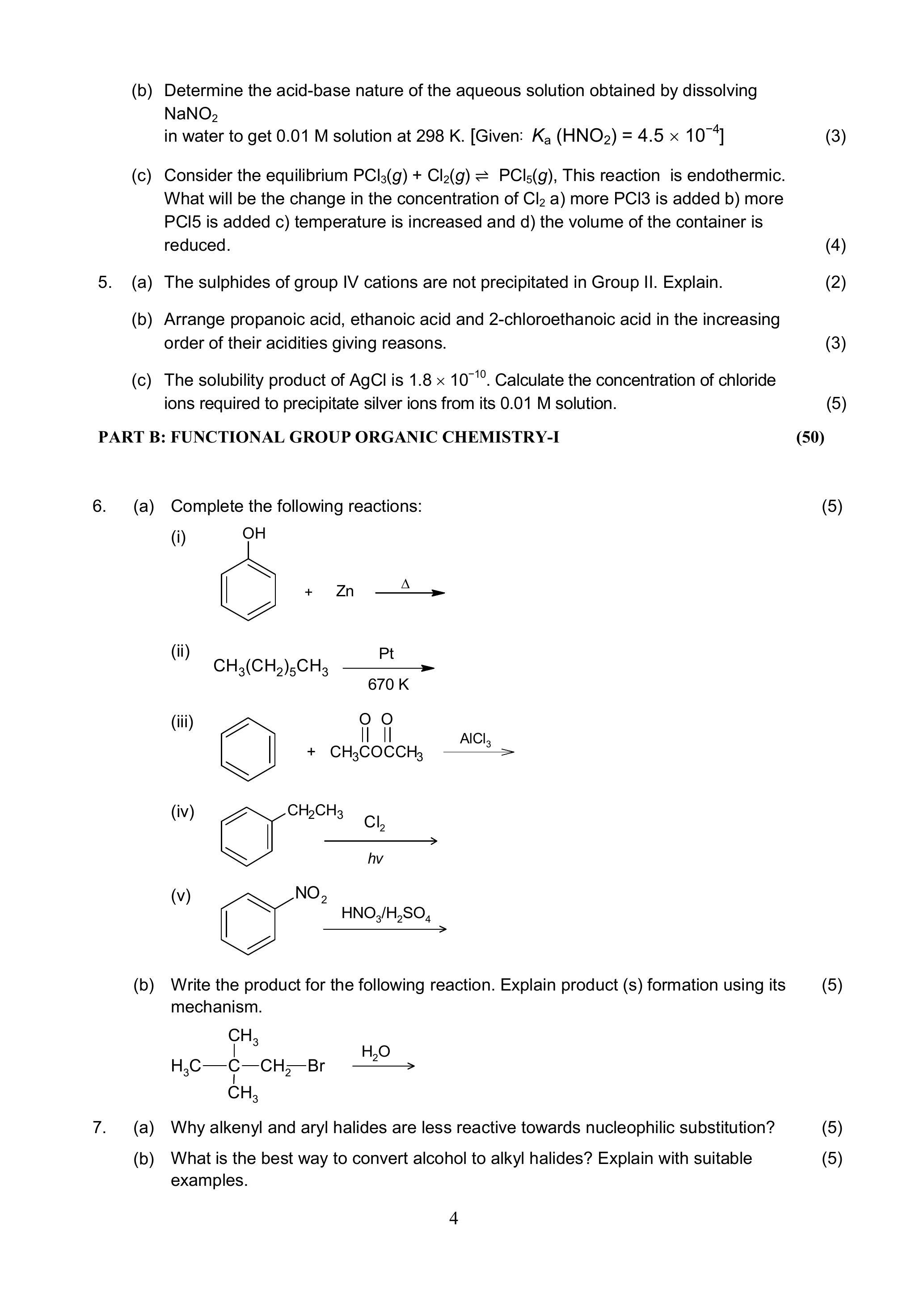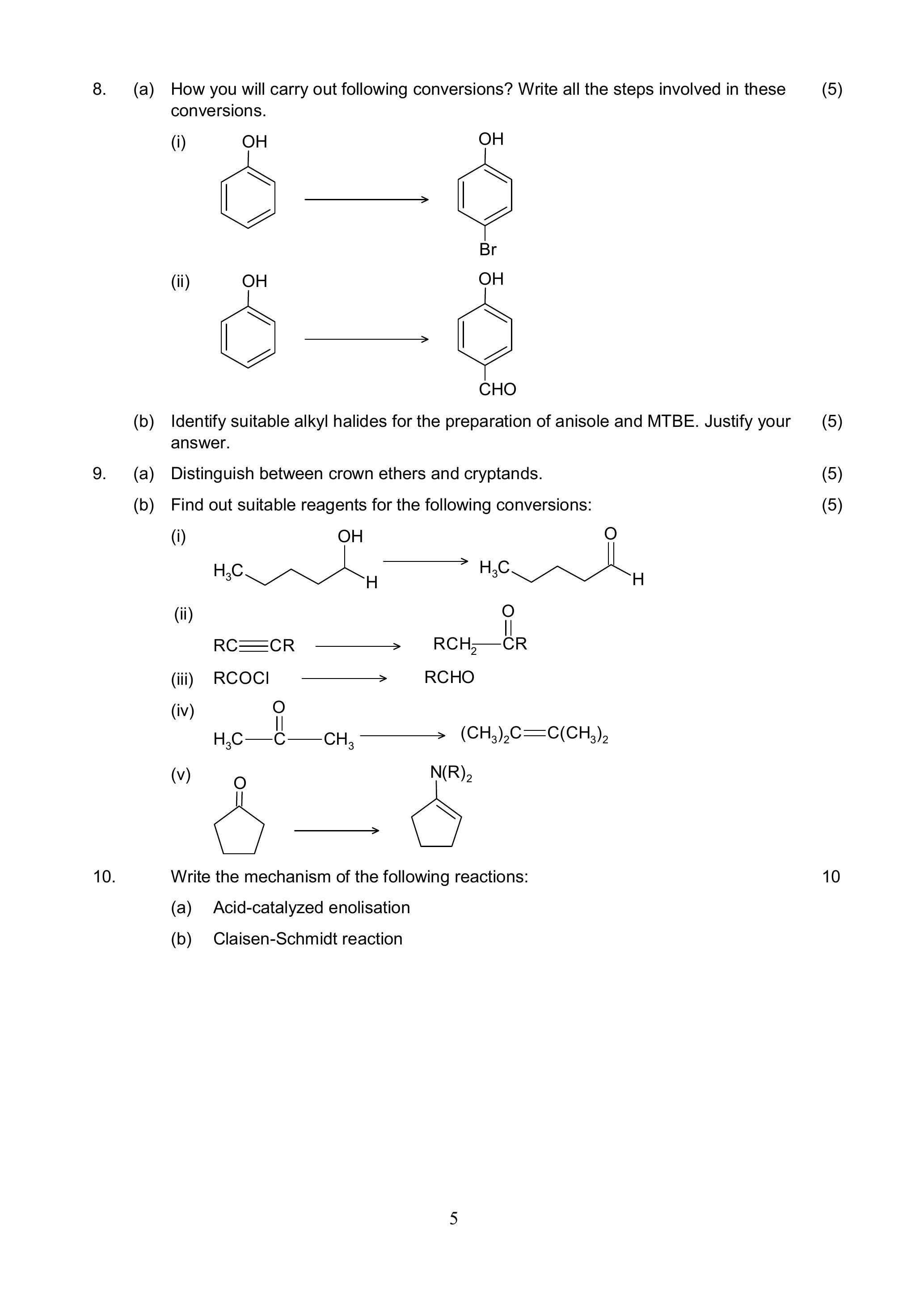# IGNOU BCHCT-133 Solved Assignment 2023 | B.Sc (G) CBCS

Solved By – Narendra Kr. Sharma – M.Sc (Mathematics Honors) – Delhi University

101.00

Details For BCHCT-133 Solved Assignment

## IGNOU BCHCT-133 Assignment Question Paper 2023

Course Code: BCHCT-133
Assignment Code: BCHCT-133/TMA/2023
Maximum Marks: 100

Note: Attempt all questions. The marks for each question are indicated against it.

PART A: CHEMICAL ENERGETICS AND EQUILIBRIA

1. (a) State Zeroth law of thermodynamics and give the requirement for thermal equilibrium.

(b) Differentiate between a closed and an isolated thermodynamic system with the help of suitable examples.

(c) 0.25 mol of an ideal monoatomic gas undergoes isothermal expansion from a volume of $$2.0 \mathrm{dm}^{3}$$ to $$10 \mathrm{dm}^{3}$$ at $$27^{\circ} \mathrm{C}$$. Calculate the maximum work that can be obtained from this process.

2. (a) Calculate the amount of heat required to increase the temperature of 2.00 moles of Argon gas from a temperature of $$200 \mathrm{~K}$$ to $$500 \mathrm{~K}$$ under isobaric conditions.

[Given: $$C_{p, \mathrm{~m}}=20.79 \mathrm{~J} \mathrm{~K}^{-1} \mathrm{~mol}^{-1}$$ ]

(b) Calculate $$\Delta H^{\circ}$$ for the reaction

$$\mathrm{CO}_{2}(\mathrm{~g})+\mathrm{H}_{2}(\mathrm{~g}) \rightarrow \mathrm{CO}(\mathrm{g})+\mathrm{H}_{2} \mathrm{O}(\mathrm{g})$$

Given: $$\Delta \mathrm{H}$$ for $$\mathrm{CO}_{2}(\mathrm{~g}), \mathrm{CO}(\mathrm{g})$$ and $$\mathrm{H}_{2} \mathrm{O}(\mathrm{g})$$ are $$-393.5,-111.31$$ and $$-241.80 \mathrm{~kJ} \mathrm{~mol}^{-1}$$ respectively.

(c) Differentiate between enthalpy driven and entropy driven reactions with the help of suitable examples.

3. (a) Define enthalpy of atomisation. The enthalpy of atomisation of graphite is found to be $$715 \mathrm{~kJ} \mathrm{mo1} 1^{-1}$$, write the thermochemical equation for the process.

(b) Derive an expression for isothermal mixing of ideal gases.

(c) Fossil fuels are being replaced with hydrogen, much of it is being derived from methane, $$\mathrm{CH}_{4}$$, in natural gas which uses the forward reaction of the equilibrium:

$$\mathrm{CH}_{4}(g)+\mathrm{H}_{2} \mathrm{O}(g) \rightleftharpoons \mathrm{CO}(g)+3 \mathrm{H}_{2}(g)$$

What will be the expression for $$K_{c}$$ for the above reaction?

4. (a) The successive ionisation constants of polyprotic acids are smaller and smaller. Explain. (b) Determine the acid-base nature of the aqueous solution obtained by dissolving $$\mathrm{NaNO}_{2}$$

in water to get $$0.01 \mathrm{M}$$ solution at $$298 \mathrm{~K}$$. [Given: $$K_{\mathrm{a}}\left(\mathrm{HNO}_{2}\right)=4.5 \times 10^{-4}$$ ]

(c) Consider the equilibrium $$\mathrm{PCl}_{3}(g)+\mathrm{Cl}_{2}(g) \rightleftharpoons \mathrm{PCl}_{5}(g)$$, This reaction is endothermic. What will be the change in the concentration of $$\mathrm{Cl}_{2}$$ a) more $$\mathrm{PCl} 3$$ is added b) more $$\mathrm{PCl} 5$$ is added c) temperature is increased and d) the volume of the container is reduced.

5. (a) The sulphides of group IV cations are not precipitated in Group II. Explain.

(b) Arrange propanoic acid, ethanoic acid and 2-chloroethanoic acid in the increasing order of their acidities giving reasons.

(c) The solubility product of $$\mathrm{AgCl}$$ is $$1.8 \times 10^{-10}$$. Calculate the concentration of chloride ions required to precipitate silver ions from its $$0.01 \mathrm{M}$$ solution.

PART B: FUNCTIONAL GROUP ORGANIC CHEMISTRY-I

6. (a) Complete the following reactions:

(i)(ii)(iii)(iv)$$(\mathrm{v})$$(b) Write the product for the following reaction. Explain product (s) formation using its mechanism.7. (a) Why alkenyl and aryl halides are less reactive towards nucleophilic substitution?

(b) What is the best way to convert alcohol to alkyl halides? Explain with suitable examples. 8. (a) How you will carry out following conversions? Write all the steps involved in these conversions.

(i)(ii)(b) Identify suitable alkyl halides for the preparation of anisole and MTBE. Justify your answer.

Distinguish between crown ethers and cryptands.

(b) Find out suitable reagents for the following conversions:

(i)(ii)(iii) $$\mathrm{RCOCl} \longrightarrow \mathrm{RCHO}$$

(iv)$$(v)$$10. Write the mechanism of the following reactions:
(a) Acid-catalyzed enolisation
(b) Claisen-Schmidt reaction

$$sin\left(\theta -\phi \right)=sin\:\theta \:cos\:\phi -cos\:\theta \:sin\:\phi$$

## BCHCT-133 Sample Solution 2023

Simply click “Install” to download and install the app, and then follow the instructions to purchase the required assignment solution. Currently, the app is only available for Android devices. We are working on making the app available for iOS in the future, but it is not currently available for iOS devices.

Yes, It is Complete Solution, a comprehensive solution to the assignments for IGNOU. Valid from January 1, 2023 to December 31, 2023.

Yes, the Complete Solution is aligned with the IGNOU requirements and has been solved accordingly.

Yes, the Complete Solution is guaranteed to be error-free.The solutions are thoroughly researched and verified by subject matter experts to ensure their accuracy.

As of now, you have access to the Complete Solution for a period of 6 months after the date of purchase, which is sufficient to complete the assignment. However, we can extend the access period upon request. You can access the solution anytime through our app.

The app provides complete solutions for all assignment questions. If you still need help, you can contact the support team for assistance at Whatsapp +91-9958288900

No, access to the educational materials is limited to one device only, where you have first logged in. Logging in on multiple devices is not allowed and may result in the revocation of access to the educational materials.

Payments can be made through various secure online payment methods available in the app.Your payment information is protected with industry-standard security measures to ensure its confidentiality and safety. You will receive a receipt for your payment through email or within the app, depending on your preference.

The instructions for formatting your assignments are detailed in the Assignment Booklet, which includes details on paper size, margins, precision, and submission requirements. It is important to strictly follow these instructions to facilitate evaluation and avoid delays.

$$sin\:3\theta =3\:sin\:\theta -4\:sin^3\:\theta$$

## Terms and Conditions

• The educational materials provided in the app are the sole property of the app owner and are protected by copyright laws.
• Reproduction, distribution, or sale of the educational materials without prior written consent from the app owner is strictly prohibited and may result in legal consequences.
• Any attempt to modify, alter, or use the educational materials for commercial purposes is strictly prohibited.
• The app owner reserves the right to revoke access to the educational materials at any time without notice for any violation of these terms and conditions.
• The app owner is not responsible for any damages or losses resulting from the use of the educational materials.
• The app owner reserves the right to modify these terms and conditions at any time without notice.
• By accessing and using the app, you agree to abide by these terms and conditions.
• Access to the educational materials is limited to one device only. Logging in to the app on multiple devices is not allowed and may result in the revocation of access to the educational materials.

Our educational materials are solely available on our website and application only. Users and students can report the dealing or selling of the copied version of our educational materials by any third party at our email address (abstract4math@gmail.com) or mobile no. (+91-9958288900).

In return, such users/students can expect free our educational materials/assignments and other benefits as a bonafide gesture which will be completely dependent upon our discretion.

Insert math as
$${}$$